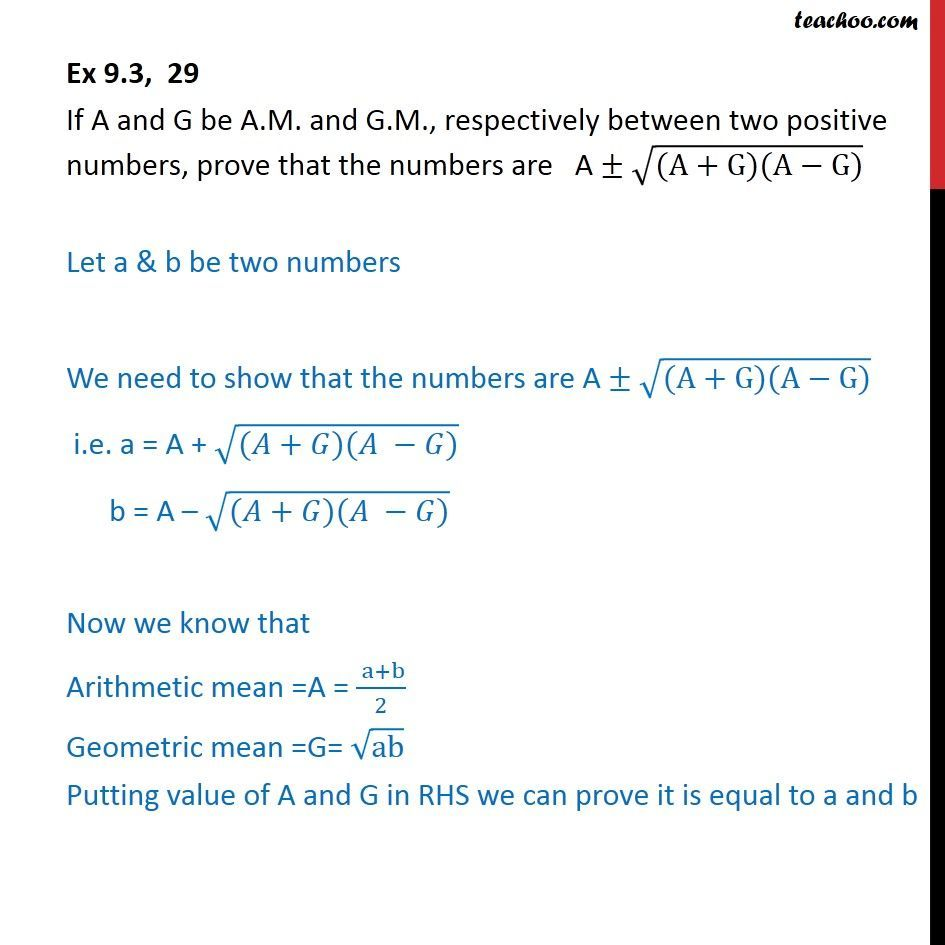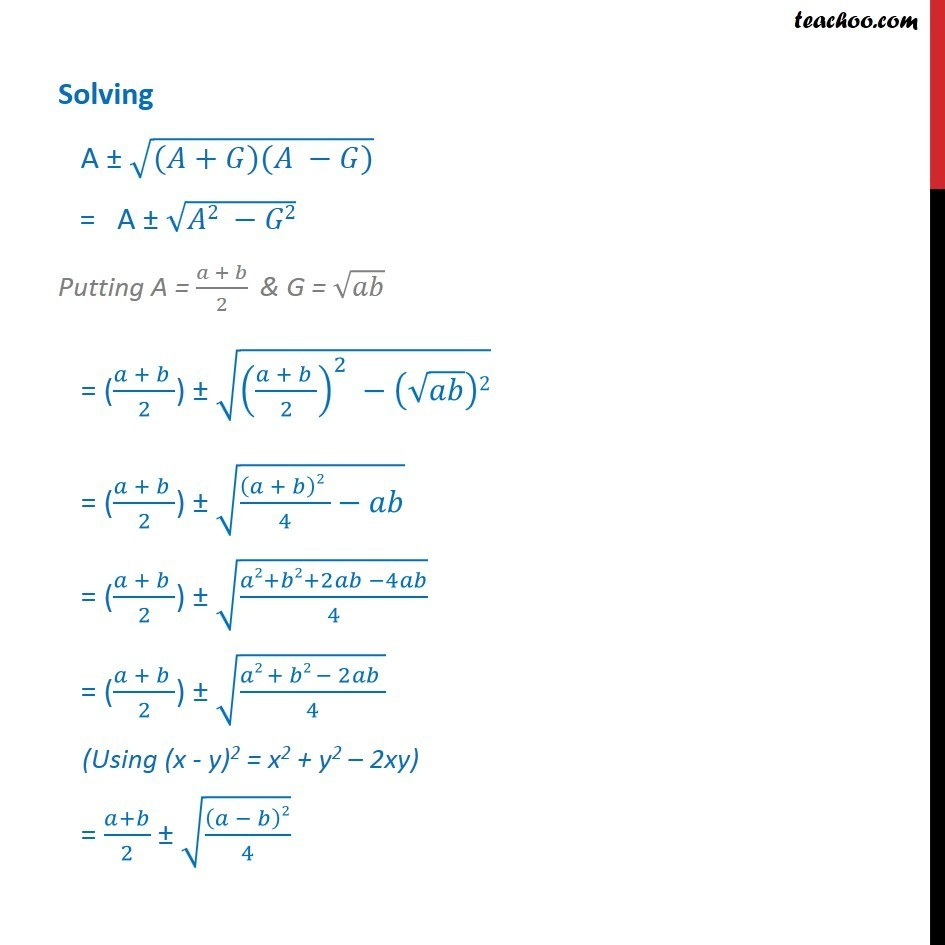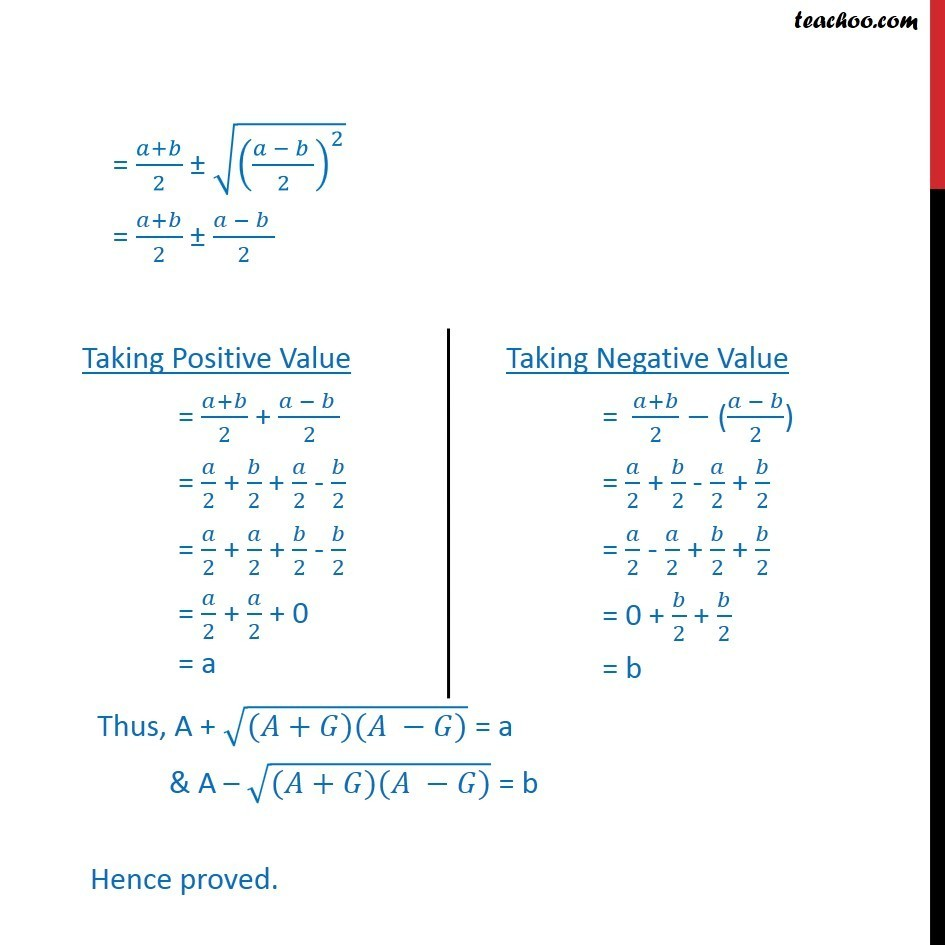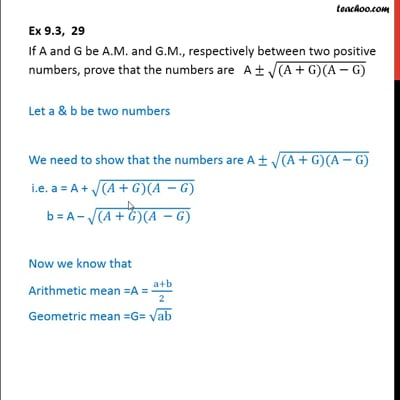Ex 9.3

Chapter 9 Class 11 Sequences and Series (Term 1)
Serial order wiseThis video is only available for Teachoo black users

### Transcript

Ex 9.3, 29 If A and G be A.M. and G.M., respectively between two positive numbers, prove that the numbers are A ((A+G)(A G)) Let a & b be two numbers We need to show that the numbers are A ((A+G)(A G)) i.e. a = A + (( + )( )) b = A (( + )( )) Now we know that Arithmetic mean =A = ( a+b)/2 Geometric mean =G= ab Putting value of A and G in RHS we can prove it is equal to a and b Solving A (( + )( )) = A ( 2 2) Putting A = ( + )/2 & G = = (( + )/2) ((( + )/2)^2 ( )2) = (( + )/2) ((( + )2 )/4 ) = (( + )/2) (( 2+ 2+2 4 )/4) = (( + )/2) (( 2 + 2 2 )/4) = ( + )/2 (( )2/4) = ( + )/2 ((( )/2)^2 ) = ( + )/2 ( )/2 Thus, A + (( + )( )) = a & A (( + )( )) = b Hence proved.